# JP4449400B2 - Image encoding apparatus and method, program, and recording medium - Google Patents

## Info

Publication number
JP4449400B2
JP4449400B2 JP2003351392A JP2003351392A JP4449400B2 JP 4449400 B2 JP4449400 B2 JP 4449400B2 JP 2003351392 A JP2003351392 A JP 2003351392A JP 2003351392 A JP2003351392 A JP 2003351392A JP 4449400 B2 JP4449400 B2 JP 4449400B2
Authority
JP
Japan
Prior art keywords
encoding
number
bit
encoding target
code
Prior art date
Legal status (The legal status is an assumption and is not a legal conclusion. Google has not performed a legal analysis and makes no representation as to the accuracy of the status listed.)
Expired - Fee Related
Application number
JP2003351392A
Other languages
Japanese (ja)
Other versions
JP2004166254A (en
JP2004166254A5 (en
Inventor

Original Assignee
ソニー株式会社
Priority date (The priority date is an assumption and is not a legal conclusion. Google has not performed a legal analysis and makes no representation as to the accuracy of the date listed.)
Filing date
Publication date
Priority to JP2002311943 priority Critical
Application filed by ソニー株式会社 filed Critical ソニー株式会社
Priority to JP2003351392A priority patent/JP4449400B2/en
Publication of JP2004166254A publication Critical patent/JP2004166254A/en
Publication of JP2004166254A5 publication Critical patent/JP2004166254A5/ja
Application granted granted Critical
Publication of JP4449400B2 publication Critical patent/JP4449400B2/en
Application status is Expired - Fee Related legal-status Critical
Anticipated expiration legal-status Critical

## Images

•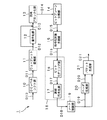•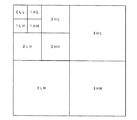•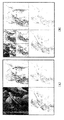•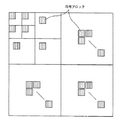•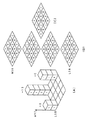•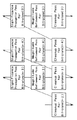•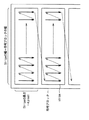•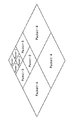•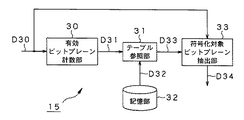•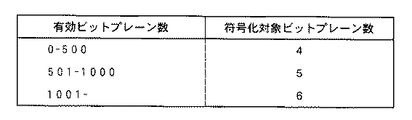•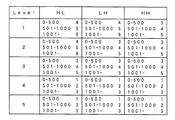•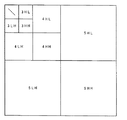•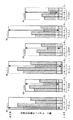•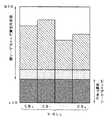•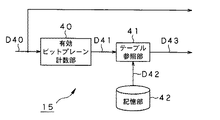•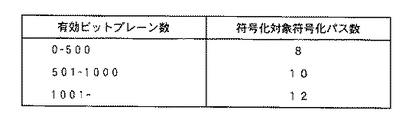•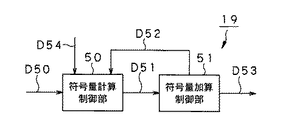•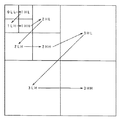•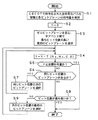•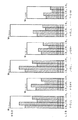•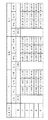•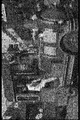•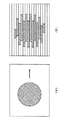•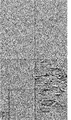•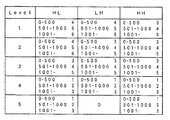## Classifications

• GPHYSICS
• G06COMPUTING; CALCULATING; COUNTING
• G06TIMAGE DATA PROCESSING OR GENERATION, IN GENERAL
• G06T9/00Image coding
• G06T9/004Predictors, e.g. intraframe, interframe coding

## Description

The present invention records, for example, an image encoding apparatus and method for compressing an image by wavelet transform and entropy encoding as in the JPEG-2000 system, a program for causing a computer to execute image encoding processing, and the program. The present invention relates to a recording medium.

As a conventional typical image compression method, there is a JPEG (Joint Photographic Experts Group) method standardized by ISO (International Standards Organization). This is known to use a discrete cosine transform (DCT) and provide a good encoded image and decoded image when relatively high bits are assigned. However, if the number of encoded bits is reduced to a certain degree, block distortion peculiar to DCT becomes remarkable, and deterioration becomes conspicuous subjectively.

On the other hand, in recent years, research on a method in which an image is divided into a plurality of bands by a filter combining a high-pass filter and a low-pass filter called a filter bank and coding is performed for each band has been actively conducted. Among them, wavelet transform coding is regarded as a promising new technology to replace DCT because it does not have the disadvantage that block distortion becomes remarkable due to high compression unlike DCT.

The JPEG-2000 system, whose international standardization was completed in January 2001, employs a system that combines this wavelet transform with highly efficient entropy coding (bit modeling and arithmetic coding in units of bit planes). Compared to this, the coding efficiency is greatly improved.

In these international standards, only the standard on the decoder side is defined, and the encoder side can be designed freely. On the other hand, since there is no standard for an effective rate control method that achieves the target compression rate, the establishment of know-how is most important.

In particular, in the JPEG method, this rate control is difficult, and it is often necessary to perform encoding a plurality of times before obtaining the target value. However, since this leads to an increase in processing time, in the JPEG-2000 system, it is desired to obtain a target code amount by one encoding.

Here, in the JPEG-2000 system, a rate control method using RD (Rate-Distortion) characteristics is generally used. However, although this rate control method is versatile, it has a very high computational load. There were drawbacks.

Therefore, the present applicant has proposed a technique for performing rate control by truncating a once generated encoded code stream from the tail in Patent Document 1 below. According to this technique, it is possible to perform control in accordance with the target code amount accurately.

JP 2002-165098 A

However, in the technique of Patent Document 1, when there are code blocks that have a large influence on image quality at the end of the encoded code stream, they may be discarded, resulting in image quality deterioration, which is more effective. A rate control technique is desired.

In addition, in the JPEG-2000 system, there is no standard for a method for reducing entropy coding processing, which is generally a heavy load, and thus establishment of know-how is most important.

Furthermore, the JPEG-2000 system is a standard for still images, and sufficient consideration has not been made for interlaced images that frequently exist in moving images. Therefore, if the technology for still images is applied to a moving image as it is, the disadvantage that image quality deterioration becomes conspicuous is exposed.

The present invention has been proposed in view of such conventional circumstances. For example, in a JPEG-2000 image encoding apparatus, the processing load of arithmetic encoding is reduced and image quality deterioration is effectively suppressed. Another object of the present invention is to provide an image encoding apparatus and method for performing rate control, a program for causing a computer to execute image encoding processing, and a computer-readable recording medium on which the program is recorded.

In order to achieve the above-described object, an image coding apparatus according to the present invention generates a subband by applying a low-pass filter and a high-pass filter to an input image in a vertical direction and a horizontal direction, and generates a low-band component. and facilities to filtering means hierarchically filtering processing on subbands divides the sub-band generated by said filtering means, a code block generating means for generating a code block of a predetermined size, the code block A bit plane generating means for generating a bit plane from the most significant bit to the least significant bit as a unit, an encoding target prediction means for predicting the encoding target number to be encoded and generating encoding target number information , among the encoding target generated by the encoding target prediction unit, the encoding target number information from the most significant bit side of each code block For given coded only encode a row Cormorant encoding means, the encoding target prediction unit, the number of effective bit-planes except the zero bit planes for all code blocks in a frame of the input image By counting and referring to a predetermined table based on the counting result, the number of encoding objects to be encoded is obtained for each frame.

In order to achieve the above-described object, the image coding method according to the present invention applies a low-pass filter and a high-pass filter to an input image in the vertical direction and the horizontal direction to generate subbands, A filtering process for hierarchically filtering the subbands of the band components, a code block generating process for generating a code block of a predetermined size by dividing the subband generated in the filtering process, and A bit plane generating step for generating a bit plane from the most significant bit to the least significant bit for each code block, and an encoding target prediction step for generating the encoding target number information by predicting the encoding target number to be encoded And among the encoding targets generated in the encoding target prediction step, the encoding target number information from the most significant bit side of each code block Erareru the encoding for the subject count only possess an encoding step of performing encoding, in the coded prediction step, the effective bit planes excluding the zero bit planes for all code blocks in a frame of the input image By counting the number and referring to a predetermined table based on the counting result, the number of encoding objects to be encoded is obtained for each frame.

In order to achieve the above-described object, the image coding apparatus according to the present invention generates a subband by applying a low-pass filter and a high-pass filter to an input image in a vertical direction and a horizontal direction to generate a subband. Filtering means for hierarchically filtering the subbands of the band components; code block generating means for dividing the subband generated by the filtering means to generate a code block of a predetermined size; and the code A bit plane generating means for generating a bit plane from the most significant bit to the least significant bit in block units, and an encoding target prediction means for predicting the number of encoding targets to be encoded and generating encoding target number information Of the encoding targets generated by the encoding target prediction means, the encoding targets from the most significant bit side of each code block An encoding unit that performs encoding only for an encoding target given by information, and the encoding target prediction unit includes effective bit planes excluding zero bit planes for all code blocks in a subband of the input image. By counting the number and referring to a predetermined table based on the counting result, the number of encoding targets to be encoded is obtained for each subband.

In order to achieve the above-described object, the image coding method according to the present invention applies a low-pass filter and a high-pass filter to an input image in the vertical direction and the horizontal direction to generate subbands, A filtering process for hierarchically filtering the subbands of the band components, a code block generating process for generating a code block of a predetermined size by dividing the subband generated in the filtering process, and A bit plane generating step for generating a bit plane from the most significant bit to the least significant bit for each code block, and an encoding target prediction step for generating the encoding target number information by predicting the encoding target number to be encoded And the encoding target number information from the most significant bit side of each code block among the encoding targets generated in the encoding target prediction step. The encoding step for encoding only the number of encoding targets obtained, and in the encoding target prediction step, the effective bits excluding zero bit planes for all the code blocks in the subband of the input image By counting the number of planes and referring to a predetermined table based on the counting result, the number of encoding targets to be encoded is obtained for each subband.

A program according to the present invention causes a computer to execute the above-described image encoding process, and a recording medium according to the present invention is a computer-readable medium on which such a program is recorded.

According to the image encoding device and the method thereof according to the present invention, the encoding target number information is generated by predicting the encoding target number to be encoded, and the most significant bit of each code block among the encoding targets By performing the encoding only on the encoding target given by the encoding target number information from the side, the processing load of the encoding can be reduced.

Moreover, according to the program and recording medium which concern on this invention, the image coding process mentioned above is realizable with software.

Hereinafter, specific embodiments to which the present invention is applied will be described in detail with reference to the drawings. In this embodiment, the present invention is applied to an image encoding apparatus and method for compressing and encoding an input image by the JPEG-2000 system. In the following, first, the overall configuration and operation of the image encoding device will be described, and then the main part to which the present invention is applied in this image encoding device will be described.

(1) Configuration and Operation of Image Encoding Device FIG. 1 shows a schematic configuration of the image encoding device in the present embodiment. As shown in FIG. 1, the image encoding device 1 includes a DC level shift unit 10, a wavelet transform unit 11, a quantization unit 12, a code block unit 13, a bit plane decomposition unit 14, and an encoding target. The prediction unit 15, the bit modeling unit 16, the arithmetic coding unit 17, the rate control unit 19, the header generation unit 20, and the packet generation unit 21 are configured. Here, the bit modeling unit 16 and the arithmetic coding unit 17 constitute an EBCOT (Embedded Coding with Optimized Truncation) unit 18.

The DC level shift unit 10 performs level shift of the original signal in order to efficiently perform the wavelet transform in the wavelet transform unit 11 in the subsequent stage and improve the compression rate. In principle, since the RGB signal has a positive value (an unsigned integer value), the compression efficiency can be improved by performing a level shift that halves the dynamic range of the original signal. On the other hand, since the color difference signals such as Cb and Cr in the YCbCr signal have both positive and negative integer values, level shift is not performed.

The wavelet transform unit 11 is usually realized by a filter bank including a low-pass filter and a high-pass filter. Since a digital filter usually has an impulse response (filter coefficient) having a multi-tap length, it is necessary to buffer an input image that can be filtered in advance. Is omitted.

The DC level shift unit 10 receives the minimum image signal D10 necessary for filtering and performs level shift as described above. Then, the wavelet transform unit 11 performs a filtering process for performing wavelet transform on the image signal D11 after the DC level shift, and generates a wavelet transform coefficient D12.

In this wavelet transform, the low frequency components are usually repeatedly transformed as shown in FIG. 2, because most of the energy of the image is concentrated on the low frequency components. This is because subbands are formed as shown in the figure as the division level is advanced from division level = 1 shown in FIG. 3A to division level = 3 shown in FIG. 3B. It can be seen from going. Here, the number of wavelet transform levels in FIG. 2 is 3, and as a result, a total of 10 subbands are formed. Here, L and H in FIG. 2 represent a low frequency and a high frequency, respectively, and the numbers before L and H represent a division level. That is, for example, 1LH represents a subband of division level = 1 in which the horizontal direction is a low frequency and the vertical direction is a high frequency.

The quantization unit 12 performs irreversible compression on the wavelet transform coefficient D12 supplied from the wavelet transform unit 11. As the quantization means, scalar quantization that divides the wavelet transform coefficient D12 by the quantization step size can be used. Here, according to the JPEG-2000 standard, when the above-mentioned lossy compression is performed and the lossy 9 × 7 wavelet transform filter is used, it is decided to automatically use scalar quantization together. Yes. On the other hand, when a reversible 5 × 3 wavelet transform filter is used, quantization is not performed and code amount control is performed in a rate control unit 19 described later. Therefore, the quantization unit 12 in FIG. 1 operates when an irreversible 9 × 7 wavelet transform filter is actually used. Hereinafter, the description will be made assuming that this irreversible 9 × 7 wavelet transform filter is used.

The code blocking unit 13 divides the quantized coefficient D13 generated by the quantizing unit 12 into code blocks having a predetermined size, which is a processing unit of entropy coding. Here, the positional relationship of the code blocks in the subband is shown in FIG. Usually, for example, a code block having a size of about 64 × 64 is generated in all subbands after division. Therefore, when the size of the 3HH subband having the smallest division level in FIG. 2 is 640 × 320, the number of 64 × 64 code blocks is 10 in the horizontal direction and 5 in the vertical direction, for a total of 50. There will be. The code block unit 13 supplies the quantization coefficient D14 for each code block to the bit plane decomposition unit 14, and the subsequent encoding process is performed for each code block.

The bit plane decomposition unit 14 decomposes the quantization coefficient D14 for each code block into bit planes. The concept of this bit plane will be described with reference to FIG. FIG. 5A assumes a quantized coefficient composed of a total of 16 coefficients of 4 vertical and 4 horizontal. Among the 16 coefficients, the coefficient having the maximum absolute value is 13, which is 1101 in binary representation. Therefore, the bit plane of the absolute value of the coefficient is composed of four bit planes as shown in FIG. Note that all the elements of each bit plane take a number of 0 or 1. On the other hand, as for the sign of the quantized coefficient, −6 is the only negative value, and other values are 0 or a positive value. Therefore, the bit plane of the code is as shown in FIG. The bit plane decomposition unit 14 supplies the quantization coefficient D15 decomposed into the bit brains to the encoding target prediction unit 15.

The encoding target prediction unit 15 uses the EBCOT unit 18 to encode the number of bit planes or the number of encoding passes to be finally encoded based on the quantization coefficient D15 decomposed into bit brains by the bit plane decomposition unit 14. Pre-prediction is performed, the predicted bit plane is extracted, and the coefficient bit D 16 for each extracted bit plane is supplied to the bit modeling unit 16. Details of the prediction process in the encoding target prediction unit 15 will be described later.

The bit modeling unit 16 performs coefficient bit modeling on the coefficient bits D16 for each bit plane supplied from the encoding target prediction unit 15 as follows, and the context D17 for each coefficient bit is converted to the arithmetic coding unit 17. To supply. The arithmetic coding unit 17 performs arithmetic coding on the context D17 for each coefficient bit, and supplies the obtained arithmetic code D18 to the rate control unit 19. In this embodiment, entropy coding called EBCOT defined by the JPEG-2000 standard will be described as an example. The EBCOT is described in detail in, for example, the document “IS0 / IEC 15444-1, Information technology-JPEG 2000, Part 1: Core coding system”. As described above, the EBCOT unit 18 is configured by the bit modeling unit 16 and the arithmetic coding unit 17.

EBCOT is a means for encoding for each block of a predetermined size while measuring the statistical amount of coefficient bits in the block, and entropy-encodes the quantized coefficient in units of code blocks. The code block is encoded independently for each bit plane in the direction from the most significant bit (MSB) to the least significant bit (LSB). The vertical and horizontal sizes of the code block are powers of 2 from 4 to 256, and sizes such as 32 × 32, 64 × 64, and 128 × 32 are usually used. The quantization coefficient is represented by an n-bit signed binary number, and bit0 to bit (n−2) represent respective bits from LSB to MSB. The remaining 1 bit is a code. The coding of the code block is performed by the three kinds of coding passes shown in the following (a) to (c) in order from the bit plane on the MSB side.
(a) Significance Propagation Pass
(b) Magnitude Refinement Pass
(c) Clean Up Pass

The order in which the three coding passes are used is shown in FIG. As shown in FIG. 6, first, the bit plane (n-2) (MSB) is encoded by a Clean Up Pass (hereinafter referred to as a CU pass as appropriate). Subsequently, each bit plane is sequentially used in the order of Significance Propagation Pass (hereinafter referred to as SP pass), Magnitude Refinement Pass (hereinafter referred to as MR pass), and Clean Up Pass. It becomes.

In practice, however, the first bit plane from the MSB side where 1 appears for the first time is written in the header, and the bit plane composed of zero coefficients (zero bit plane) is not encoded. In this order, encoding is performed by repeatedly using three types of encoding passes, and the encoding is terminated up to an arbitrary encoding pass of an arbitrary bit plane, thereby taking a trade-off between code amount and image quality, that is, rate control is performed. be able to.

Here, scanning (scanning) of coefficient bits will be described with reference to FIG. The code block is divided into stripes every four coefficient bits in height. The width of the stripe is equal to the width of the code block. The scan order is the order in which all the coefficient bits in one code block are traced. In the code block, the order is from the upper stripe to the lower stripe, and in each stripe, the order is from the left column to the right column. In each row, scanning is performed from top to bottom. In each coding pass, all coefficient bits in the code block are processed in this scan order.

Hereinafter, the three coding passes described above will be described. Note that these three coding passes are all described in the above-mentioned document “IS0 / IEC 15444-1, Information technology-JPEG 2000, Part 1: Core coding system”.

(a) Significance Propagation Pass
In the SP pass for encoding a bit plane, non-significant coefficient bits in which at least one coefficient in the vicinity of 8 is significant are arithmetically encoded. When the value of the encoded coefficient bit is 1, the sign of the sign is continued and arithmetic coding is performed.

Here, the significance is a state that the encoder has for each coefficient bit. The initial value of significance is “0” representing non-significant, changes to “1” representing significant when 1 is encoded with the coefficient, and continues to be always “1” thereafter. Therefore, the significance can also be said to be a flag indicating whether or not valid digit information has already been encoded. If an SP path is generated in a certain bit plane, no SP path is generated in the subsequent bit planes.

(b) Magnitude Refinement Pass
In the MR pass that encodes the bit plane, significant coefficient bits that are not encoded in the SP pass that encodes the bit plane are arithmetically encoded.

(c) Clean Up Pass
In the CU pass that encodes the bit plane, non-significant coefficient bits that are not encoded in the SP pass that encodes the bit plane are arithmetically encoded. When the value of the encoded coefficient bit is 1, the sign of the sign is continued and arithmetic coding is performed.

In arithmetic coding in the above three coding passes, ZC (Zero Coding), RLC (Run-Length Coding), SC (Sign Coding), and MR (Magnitude Refinement) are used properly according to the case. Context is selected. Then, the selected context is encoded by an arithmetic code called MQ encoding. This MQ coding is a learning type binary arithmetic code defined by JBIG2. The MQ coding is described in, for example, the document “ISO / IEC FDIS 14492,“ Lossy / Lossless Coding of Bi-level Images ”, March 2000”. In JPEG-2000, there are a total of 19 contexts in all coding passes.

As described above, the bit modeling unit 16 processes the coefficient bit D16 for each bit plane in three coding passes, and generates a context D17 for each coefficient bit. The arithmetic encoding unit 17 performs arithmetic encoding on the context D17 for each coefficient bit.

The rate control unit 19 counts the code amount of the arithmetic code D18 supplied from the arithmetic coding unit 17 after performing at least a part of the coding pass processing, and when the target code amount is reached, The arithmetic code D18 after that is discarded. Thus, by truncating immediately before the code amount is exceeded, the target code amount can be reliably suppressed. The rate control unit 19 supplies the arithmetic code D19 after completion of the code amount control to the header generation unit 20 and the packet generation unit 21. Details of the rate control process in the rate control unit 19 will be described later.

Based on the arithmetic code D19 after completion of the code amount control supplied from the rate control unit 19, the header generation unit 20 adds additional information in the code block, for example, the number of coding paths in the code block and the compression code stream. The data length or the like is generated as a header D20, and this header D20 is supplied to the packet generator 21.

The packet generator 21 generates a packet D21 by combining the arithmetic code D19 after completion of the code amount control and the header D20, and outputs the packet D21 as an encoded code stream. At this time, the packet generator 21 generates individual packets from the same resolution level as shown in FIG. As can be seen from FIG. 8, packet-1 which is the lowest band includes only the LL component, and other packets-2 to packet-4 include the LH component, the HL component, and the HH component.

As described above, the image encoding device 1 according to the present embodiment can compress and encode an input image with high efficiency using wavelet transform and entropy encoding, packetize it, and output it as an encoded code stream.

(2) Application Part in Image Encoding Device (2-1) Configuration and Operation of Encoding Target Prediction Unit By the way, in an image encoding device that generally compresses and encodes an input image by the JPEG-2000 method, entropy encoding in EBCOT The processing load is very high. On the other hand, when encoding at a target compression rate or bit rate, there are some that are not actually used after encoding with EBCOT, and as a result, they are wasted.

Therefore, the encoding target prediction unit 15 according to the present embodiment predicts a bit plane having a high probability of being finally used, extracts only this bit plane, and supplies the extracted bit plane to the EBCOT unit 18 described above. Specifically, the number of effective bit planes for one frame or one subband is counted, and this number of effective bit planes is used as an index for the amount of code for generating the number of effective bit planes. Predict.

An example of the internal configuration of the encoding target prediction unit 15 is shown in FIG. As illustrated in FIG. 9, the encoding target prediction unit 15 refers to the effective bit plane counting unit 30 that counts the number of effective bit planes and the bit plane number table, and calculates the encoding target from the number of effective bit planes. Based on the table reference unit 31 that predicts the number of bit planes to be stored, the storage unit 32 that stores the bit plane number table, and the number of bit planes to be encoded, the encoding target bit plane is extracted as described later. The encoding target bit plane extraction unit 33 is configured. As the storage unit 32, a read only memory (ROM) that is a read-only nonvolatile storage medium can be used.

The valid bit plane counting unit 30 receives the bit plane D30 for each code block, and counts the number of valid bit planes excluding the bit plane composed of zero coefficients (zero bit plane). Further, the effective bit plane counting unit 30 adds the effective bit plane numbers of all code blocks in the frame or subband, and supplies the calculated total sum D 31 of effective bit planes to the table reference unit 31.

The table reference unit 31 refers to the bit plane number table stored in the storage unit 32, and reads out the encoding target bit plane number D32 from the sum D31 of the effective bit plane numbers supplied from the effective bit plane counting unit 30. The read encoding target bit plane number D33 is supplied to the encoding target bit plane extraction unit 33.

Here, the bit plane number table is a table in which the range of the number of effective bit planes is associated with the number of bit planes to be encoded in the range.

For example, when the total number of effective bit planes for each frame is used, as shown in FIG. 10, the number of encoding target bit planes corresponds to the range of the effective bit plane number. In this case, if the sum of the number of effective bit planes in the coding block in one frame is “600”, the number “5” is immediately set as the number of bit planes to be encoded by referring to this bit plane number table. Is obtained.

On the other hand, when the sum of the number of effective bit planes for each subband is used, the number of encoding target bitplanes corresponds to the range of the number of effective bitplanes in each subband, as shown in FIG. Become. Here, FIG. 11 shows an example of a table when the wavelet transform / division is performed five times as shown in FIG. In this case, if the sum of the number of effective bit planes in the LH subband is “100” at the division level = 3, the number of bit planes to be encoded is “3” immediately by referring to this bit plane number table. "Is obtained. The same applies to the other subbands. Note that the bit plane number table in FIG. 11 can perform finer control than the bit plane number table in FIG. 10, and the number of effective bit planes can be predicted with higher accuracy.

Returning to FIG. 9, the encoding target bit plane extraction unit 33, based on the encoding target bit plane number D33 supplied from the table reference unit 31, only the encoding target bit plane D34 from the bit plane D30 for each code block. To extract. Specifically, bit planes corresponding to the number of encoding target bit planes are extracted from the most significant bit (MSB) side for each code block. Then, the encoding target bit plane extraction unit 33 supplies the extracted encoding target bit plane D34 to the bit modeling unit 16 illustrated in FIG.

Since only the extracted encoding target bit plane D34 is actually encoded by the EBCOT unit 18 at the subsequent stage, the number of extracted encoding target bit planes D34 is greater than the number of bit planes D30 for each original code block. If less, the processing load can be reduced by that amount, and the time spent for encoding can be shortened.

In addition, since the encoding target prediction unit 15 in the present embodiment only refers to the bit plane number table in which the number of effective bit planes and the number of encoding target bit planes are associated in advance, only this table is stored. The memory capacity is sufficient, and the processing load is extremely small.

The above processing will be specifically described from the viewpoint of the bit plane with reference to FIG. FIG. 13 illustrates a bit plane for each code block (CB) in each subband for three components of Y (luminance), U, and V (color difference). For example, V-5HH at the right end indicates a bit plane for each code block (CB) existing in 5HH of the color difference component V, that is, in a subband of division level = 5 in which the horizontal direction and the vertical direction are high frequencies. In FIG. 13, a blank area indicates a zero bit plane, a hatched area indicates a bit plane that is finally included in the encoded code stream, and a dot area indicates a bit plane that is not used as a result of rate control in the subsequent stage. Note that the number of bit planes combining the shaded area and the dot area corresponds to the number of effective bit planes.

As described above, the encoding target prediction unit 15 determines the number of encoding target bit planes from the total number of effective bit planes in all code blocks for each frame or subband. For each code block (CB), bit planes corresponding to the number of bit planes to be encoded are extracted from the most significant bit (MSB) side.

A boundary line in the point area indicates a boundary between a bit plane extracted by the encoding target prediction unit 15 and a bit plane not extracted. That is, the bit plane existing on the least significant bit (LSB) side of this boundary line is not extracted by the encoding target prediction unit 15.

FIG. 14 is a diagram paying attention to Y-0LL of FIG. 13, that is, the lowest band of the luminance component Y. In FIG. 14, the black area is a bit plane that can be omitted in the EBCOT unit 18 as a result.

In this example, the number of bit planes to be encoded is constant for all the code blocks in the same subband for each component, but the present invention is not limited to this, and for each component or code block It is also possible to variably set the number of encoding target bit planes. However, in this case, a bit plane number table in which the number of encoding target bit planes is set for each component or code block is required.

In the above description, the number of encoding target bitplanes is predicted from the number of effective bitplanes by referring to the bitplane number table, but the number of encoding target encoding passes is predicted from the number of effective bitplanes. It doesn't matter if you do.

FIG. 15 shows an internal configuration of the encoding target prediction unit 15 in this case. As shown in FIG. 15, the encoding target prediction unit 15 refers to the effective bit plane counting unit 40 that counts the number of effective bit planes and the bit plane number table described above, and determines the encoding target from the number of effective bit planes. The table reference unit 41 obtains the number of encoding passes and the storage unit 42 stores the encoding pass number table.

The effective bit plane counting unit 40 inputs the bit plane D40 for each code block, counts the number of effective bit planes, and adds the number of effective bit planes of all code blocks in the frame or subband. Is supplied to the table reference unit 41.

The table reference unit 41 refers to the encoding pass number table stored in the storage unit 42 and calculates the encoding target encoding pass number D42 from the sum D41 of the effective bit plane numbers supplied from the effective bit plane counting unit 40. And the read encoding target encoding pass number D43 is supplied to the bit modeling unit 16 shown in FIG.

Here, the encoding pass number table associates the range of the number of effective bit planes with the number of encoding target encoding passes in the range.

For example, when the total number of effective bit planes for each frame is used, the number of encoding target encoding passes corresponds to the range of the number of effective bit planes as shown in FIG. As can be seen from the comparison with FIG. 10, since a maximum of three coding passes may occur in one bit plane, the number is larger than the number of bit planes to be coded in FIG. It is set.

Since the EBCOT unit 18 at the subsequent stage actually encodes only the number of encoding passes corresponding to the number of encoding target encoding passes from the bit plane on the most significant bit (MSB) side for each code block, If the number of encoding passes D43 is smaller than the number of encoding passes generated in the bit plane D40 for each original code block, the processing load can be reduced correspondingly, and the time spent for encoding can be reduced.

(2-2) Configuration and Operation of Rate Control Unit When the arithmetic code D18 supplied from the arithmetic coding unit 17 in FIG. 1 goes directly to the subsequent stage without passing through the rate control unit 19, the target compression rate or bit There is a high probability that non-rate coded code streams will be discharged. Accordingly, the rate control unit 19 needs to perform final rate control.

Here, the rate control unit 19 in the present embodiment selects arithmetic codes in descending order of priority, adds the code amounts, and stops when the target code amount is reached.

An example of the internal configuration of the rate control unit 19 is shown in FIG. As shown in FIG. 17, the rate control unit 19 includes a code amount calculation control unit 50 and a code amount addition control unit 51.

The code amount calculation control unit 50 calculates the code amount D51 for each bit plane of the arithmetic code D50 supplied from the arithmetic encoding unit 17 (FIG. 1), and supplies the code amount D51 to the code amount addition control unit 51. The code amount addition control unit 51 adds the code amount D51 for each bit plane according to the priority order described later. The added bit plane information D52 is supplied again to the code amount calculation control unit 50, and the code amounts of all the bit planes added so far are compared with the target code amount D54. Then, when the target code amount D54 is reached, this loop control is terminated, and the arithmetic code D53 after the final code amount control is supplied to the header generation unit 20 and the packet generation unit 21 shown in FIG.

Here, the priority order of adding the code amount D51 for each bit plane is as follows. That is, among the most significant bit (MSB) bit planes of all the subbands, the bit plane having the highest bit position between the subbands is selected in order from the least significant bit (LSB) bit plane.

In addition, bit planes at the same bit position in each subband are selected in the order of the lowest subband to the highest subband. For example, when wavelet transform / division is performed three times as shown in FIG. 2, selection is made in the order of 0LL, 1HL, 1LH, 1HH, 2HL, 2LH, 2HH, 3HL, 3LH, and 3HH as shown in FIG. This is because important parts of the image are gathered in the low range rather than the high range.

Further, bit planes at the same bit positions of the three components Y (luminance), U, and V (color difference) are selected in the order of Y, U, and V, for example. This is because human visual characteristics are generally more sensitive to luminance information than color difference information. Since the importance of U and V, which are the same color difference information, depends on the input image, it is preferable to change the priority appropriately.

This bit plane addition processing procedure is shown in the flowchart of FIG. First, in step S1, information on all coding passes encoded by the EBCOT unit 18 and the code amount of each bit plane are held.

Next, in step S2, the added code amount Y is initialized to 0, and in the subsequent step S3, the first bit plane having the highest bit position between subbands including the zero bit plane is selected. Here, when there are a plurality of bit planes at the same bit position, as described above, for the subbands, for example, Y, U, and V for components in the order of the lowest band to the highest band. Select in order.

In step S4, the code amount T [Ns, Nc, C, B] of the selected bit plane is added to Y. Here, Ns, Nc, C, and B indicate a subband number, a component number, a code block number, and a bit plane number, respectively.

In step S5, it is determined whether or not the added code amount Y is greater than or equal to the target code amount. When the added code amount Y is equal to or greater than the target code amount (Yes), the addition process is terminated, and when the code amount Y is less than the target code amount (No), the process proceeds to step S6.

In step S6, it is determined whether there is a bit plane at the same bit position. If there is a bit plane at the same bit position (Yes), the process proceeds to step S7, the next bit plane is selected, and the process returns to step S4. On the other hand, if there is no bit plane at the same bit position (No), the process proceeds to step S8.

In step S8, it is determined whether or not the bit position is the lowest, that is, the least significant bit (LSB). If the bit position is the lowest (Yes), the addition process is terminated. If not (No), the first bit plane of the next bit position on the least significant bit (LSB) side is selected in Step S9 and the step is performed. Return to S4.

The above processing will be specifically described from the viewpoint of the bit plane with reference to FIG. FIG. 20 illustrates a bit plane for each coding block (CB) in each subband for three components of Y (luminance), U, and V (color difference). As in FIG. 13, the blank area indicates a zero bit plane, the hatched area indicates a bit plane that is finally included in the encoded code stream, and the dot area indicates a bit plane that is not used as a result of rate control.

As shown in FIG. 20, in the rate control unit 19, subbands are arranged in the order from the bit plane having the highest bit position including the zero bit plane to the bit plane having the lowest bit position, that is, the bit plane of the least significant bit (LSB). In the same bit position, bit planes are selected in the order of the lowest band to the highest band, and the components in the order of Y, U, V, for example. Specifically, in FIG. 20, since the bit positions of Y-0LL, U-0LL, and V-0LL are the highest among subbands, the most significant bit (MSB) in the order of Y-0LL, U-0LL, and V-0LL. The bit plane is selected from the () side.

In this example, Y-0LL to Y-5HH, U-0LL to U-5HH, V-0LL to V-5HH, and the selection for each subband are given priority over the selection for each component. The selection is not limited, and selection for each component may be given priority over selection for each subband.

As described above, according to the rate control unit 19 in the present embodiment, the number of bit planes to be discarded without being finally selected is the least significant bit (LSB) side for all code blocks in one frame. Since only one bit plane is different at the maximum, there is no difference in image quality between subbands, and an overall high-quality image can be obtained.

(2-3) Operation of Quantization Unit As described above, the quantization unit 12 is irreversible by scalar quantization that divides the wavelet transform coefficient D12 supplied from the wavelet transform unit 11 by the quantization step size. Apply compression.

By the way, the quantization defined in the JPEG-2000 standard is a conversion coefficient a b (x, y) (where x indicates the position in the horizontal direction and y is The quantization coefficient Q b (x, y) is calculated by dividing (vertical position) by the quantization step size ΔW b of the subband b.

ΔW b in equation (1) can be calculated according to equation (2) below. Here, ΔB-Step indicates a basic step size common to all subbands, L2 b indicates the L2 norm of the combined filter base waveform of subband b , and ΔN b indicates normalized quantization of subband b. Indicates the step size.

Next, after ΔW b is calculated, an index ε b and a mantissa μ b are obtained according to the following equation (3). Here, R b is the dynamic range in subband b. These values ε b and μ b are actually included in the final encoded code stream.

Here, the quantization unit 12 according to the present embodiment uses the weighting coefficient VW b for each subband b instead of using the above-described equation (2) when obtaining the quantization step size ΔW b . It calculates according to the following formula | equation (4).

The weighting factor VW b are each subband, or Y (luminance), Cb, can be set for each component such as Cr (color difference) is stored as the weighting coefficient table. When the value of the weighting factor is large, ΔW b is reduced from the above-described equation (3), and the quantization coefficient Q b (x, y) is increased from the equation (1). It is preferable to increase the value as the low frequencies are concentrated. Further, since the human visual characteristic is more sensitive to the luminance information (Y) than the color difference information (Cb, Cr), it is preferable to increase the value of the luminance information (Y).

FIG. 21 shows an example of a weighting coefficient table in the case where the wavelet transformation / division is performed five times as shown in FIG. As shown in FIG. 21, the value is larger as the division level is lower, and the luminance information (Y) is larger than the color difference information (Cb, Cr).

In the code block generation unit 13 at the subsequent stage, the quantization coefficient D13 generated by the quantization unit 12 is divided into code blocks of a predetermined size, and in the bit plane decomposition unit 14, the quantization coefficient D14 for each code block is a bit. Since it is decomposed into planes, the number of bit planes increases as the value of the quantization coefficient increases. Therefore, as described above, when the rate control unit 19 selects the arithmetic code for each bit plane or for each coding pass in the order from the arithmetic code having the highest bit position to the arithmetic code of the least significant bit in all code blocks. Therefore, more important information can be preferentially included in the encoded code stream, and as a result, a high-quality encoded image can be provided.

(3) Others The above-mentioned JPEG-2000 standard is a standard for still images, and sufficient consideration has not been made for interlaced images that frequently exist in moving images. Therefore, if the technology for still images is applied to a moving image as it is, the disadvantage that the deterioration becomes conspicuous is exposed. This will be specifically described below.

One frame of a certain interlaced moving image scene is shown in FIG. When this is viewed on a progressive monitor such as a PC monitor, the influence of interlacing is strong on the moving part, and a striped pattern appears in the horizontal direction.

That is, as shown in FIG. 23A, when a round object moves in the right direction on the screen, there is no problem in the interlaced screen, but in the progressive screen, a stripe pattern is formed in the horizontal direction as shown in FIG. I can see it. This is because the interlaced screen uses interlaced scanning in odd and even fields.

FIG. 24 shows the state of each subband after the image shown in FIG. 22 is wavelet transformed and divided five times. As can be seen from FIG. 24, the energy of the subband coefficients of 5 LH components, that is, the division level = 5 in which the horizontal direction is the low frequency and the vertical direction is the high frequency is larger than the other subbands.

Therefore, if an encoded block in the 5LH subband is encoded as it is, encoding with an emphasis on the interlace component can be realized.

On the other hand, in the case of outputting to a progressive image display device, a code block in a 5 LH subband may not be encoded. Specifically, the number of bit planes or the number of coding passes of all code blocks in the 5LH subband may be set to zero. The division level is not limited to the LH component in the highest range, and the bit plane or the number of coding passes of the code block of the LH component may be set to 0 at all division levels.

For example, as shown in FIG. 11, when the encoding target prediction unit 15 predicts the number of encoding target bit planes from the total number of effective bit planes of all the code blocks for each subband, as shown in FIG. Thus, the number of encoding target bit planes corresponding to the 5LH component may be set to 0.

It should be noted that the present invention is not limited to the above-described embodiments, and various modifications can be made without departing from the scope of the present invention.

For example, in the above-described embodiment, the hardware configuration has been described. However, the present invention is not limited to this, and arbitrary processing may be realized by causing a CPU (Central Processing Unit) to execute a computer program. Is possible. In this case, the computer program can be provided by being recorded on a recording medium, or can be provided by being transmitted via the Internet or another transmission medium.

According to the above-described present invention, for example, in a JPEG-2000 image coding apparatus, it is possible to reduce the processing load of arithmetic coding and to effectively perform rate control while suppressing image quality deterioration.

It is a figure explaining schematic structure of the image coding apparatus in this Embodiment. It is a figure explaining the subband at the time of performing wavelet transformation and a division | segmentation to division | segmentation level = 3. It is a figure explaining the subband at the time of carrying out wavelet transform and a division | segmentation of an actual image. It is a figure explaining the relationship between a code block and a subband. FIG. 4A is a diagram for explaining a bit plane. FIG. 1A shows a quantized coefficient consisting of a total of 16 coefficients, FIG. 2B shows a bit plane of an absolute value of the coefficient, and FIG. ) Indicates a bit plane of the code. It is a figure explaining the process sequence of the encoding pass in a code block. It is a figure explaining the scan order of the coefficient in a code block. It is a figure explaining the packet produced | generated with the image coding apparatus. It is a figure explaining an example of an internal structure of the encoding object prediction part in the image coding apparatus. It is a figure which shows an example of the bit plane number table in the case of utilizing the sum total of the number of effective bit planes for every frame. It is a figure which shows an example of the bit plane number table in the case of utilizing the sum total of the number of effective bit planes for every subband. It is a figure explaining the subband at the time of performing wavelet transformation and a division | segmentation to division | segmentation level = 5. It is a figure which demonstrates the process in the encoding object prediction part concretely from a bit-plane viewpoint. It is a figure which paid its attention to the subband of the lowest region of the luminance component Y. It is a figure explaining an example of an internal structure of the encoding object prediction part in the case of estimating the encoding object encoding pass number. It is a figure which shows an example of the encoding pass number table in the case of utilizing the sum total of the number of effective bit planes for every subband. It is a figure explaining an example of an internal structure of the rate control part in the image coding apparatus. It is a figure explaining the selection order for every subband. It is a flowchart explaining the addition processing procedure of the code amount for every bit plane in the same rate control part. It is a figure which demonstrates the process in the rate control part concretely from a bit-plane viewpoint. It is a figure which shows an example of the weighting coefficient table in the case of weighting and quantizing for every subband. It is a figure explaining an example of an interlace moving image. FIG. 4A is a diagram for explaining how a subject image appears in an interlaced image. FIG. 3A shows a case where an object moves in the right direction on the screen, and FIG. 3B shows the object on the progressive screen. Show how it looks. It is a figure explaining the subband at the time of carrying out wavelet transform and a division | segmentation of an interlaced image. It is a figure which shows an example of the bit plane number table in case an input image is an interlace image and uses the sum total of the number of effective bit planes for each subband.

Explanation of symbols

DESCRIPTION OF SYMBOLS 1 Image coding apparatus, 10 DC level shift part, 11 Wavelet transformation part, 12 Quantization part, 13 Code block part, 14 Bit plane decomposition part, 15 Encoding object prediction part, 16 bit modeling part, 17 Arithmetic coding Unit, 18 EBCOT unit, 19 rate control unit, 20 header generation unit, 21 packet generation unit, 30 effective bit plane counting unit, 31 table reference unit, 32 storage unit, 33 encoding target bit plane extraction unit, 40 effective bit plane Counting unit, 41 Table reference unit, 42 Storage unit, 50 Code amount calculation control unit, 51 Code amount addition control unit

## Claims (17)

1. Filtering means for generating a subband by applying a low-pass filter and a high-pass filter to an input image in a vertical direction and a horizontal direction, and performing a hierarchical filtering process on the subband of the low-frequency component;
Code block generation means for dividing the subband generated by the filtering means and generating a code block of a predetermined size;
Bit plane generating means for generating a bit plane from the most significant bit to the least significant bit in the code block unit;
Encoding target prediction means for predicting the encoding target number to be encoded and generating encoding target number information;
Among the encoding targets generated by the encoding target prediction means, an encoding means for performing encoding only for the encoding target given by the encoding target number information from the most significant bit side of each code block; Prepared,
The encoding target prediction means counts the number of effective bit planes excluding zero bit planes for all code blocks in the frame of the input image, and refers to a predetermined table on the basis of the counting result for each frame. An image encoding device that obtains the number of encoding objects to be encoded.
2.   2. The image encoding device according to claim 1, wherein the number of encoding targets is the number of bit planes or the number of encoding passes to be encoded.
3.   2. The image encoding device according to claim 1, wherein the predetermined table is a table in which a range of effective bit planes and the number of encoding targets to be encoded are associated with each other.
4.   The code amount of the arithmetic code is added for each bit plane in the order of the arithmetic code having the highest bit position to the arithmetic code of the least significant bit in all the code blocks of the input image, and exceeds a predetermined target code amount. The image coding apparatus according to claim 1, further comprising code amount control means for stopping addition of the code amount of the arithmetic code.
5.   5. The image encoding device according to claim 4, wherein the code amount control means adds the code amount of the arithmetic code in the order of the lowest band to the highest band for the arithmetic code at the same bit position.
6.   5. The image coding apparatus according to claim 4, wherein the code amount control means adds the code amount of the arithmetic code in the order of the luminance information component to the color difference information component for the arithmetic code at the same bit position.
7.   Further comprising: a quantization unit that quantizes the transform coefficient in the subband generated by the filtering unit by dividing the transform coefficient by a quantization step size that is weighted using a weighting factor set for each subband. Item 2. The image encoding device according to Item 1.
8.   The image coding apparatus according to claim 7, wherein the weighting factor is set such that the quantization step size becomes smaller as a subband of a low frequency component.
9.   8. The image encoding apparatus according to claim 7, wherein the weighting coefficient is set so that the quantization step size is smaller in the luminance information component than in the color difference information component.
10. Filtering means for generating a subband by applying a low-pass filter and a high-pass filter to an input image in a vertical direction and a horizontal direction, and performing a hierarchical filtering process on the subband of the low-frequency component;
Code block generation means for dividing the subband generated by the filtering means and generating a code block of a predetermined size;
Bit plane generating means for generating a bit plane from the most significant bit to the least significant bit in the code block unit;
Encoding target prediction means for predicting the encoding target number to be encoded and generating encoding target number information;
Among the encoding targets generated by the encoding target prediction means, an encoding means for performing encoding only for the encoding target given by the encoding target number information from the most significant bit side of each code block; Prepared,
The encoding target prediction unit counts the number of effective bit planes excluding zero bit planes for all code blocks in the subband of the input image, and refers to a predetermined table based on the counting result, thereby An image encoding apparatus that obtains the number of encoding targets for each band.
11. The image coding apparatus according to claim 10 , wherein the predetermined table is a table in which a range of effective bit planes and the number of coding targets to be coded are associated with each subband.
12. If the input image is an interlaced image, the horizontal direction according to claim 11, wherein the division level in the low range is vertical high frequency is the coded number corresponding to the subband of the highest region is set to 0 Image encoding device.
13. If the input image is an interlaced image, the horizontal direction according to claim 11, wherein said coded number corresponding to the subbands of all the division level is high band in the vertical direction in the low range is set to 0 the image Encoding device.
14. A filtering step of applying a low-pass filter and a high-pass filter to the input image in a vertical direction and a horizontal direction to generate subbands, and hierarchically filtering the subbands of the low-frequency components;
A code block generation step of dividing the subband generated in the filtering step and generating a code block of a predetermined size;
A bit plane generating step for generating a bit plane from the most significant bit to the least significant bit in the code block unit;
An encoding target prediction step for predicting the encoding target number to be encoded and generating encoding target number information;
Encoding that performs encoding only for the number of encoding targets given by the number of encoding targets information from the most significant bit side of each code block among the encoding targets generated in the encoding target prediction step A process,
In the encoding target prediction step, the number of effective bit planes excluding zero bit planes is counted for all code blocks in the frame of the input image, and a predetermined table is referred to based on the counting result, so that An image encoding method for obtaining the number of encoding objects to be encoded.
15. A filtering step of applying a low-pass filter and a high-pass filter to the input image in a vertical direction and a horizontal direction to generate subbands, and hierarchically filtering the subbands of the low-frequency components;
A code block generation step of dividing the subband generated in the filtering step and generating a code block of a predetermined size;
A bit plane generating step for generating a bit plane from the most significant bit to the least significant bit in the code block unit;
An encoding target prediction step for predicting the encoding target number to be encoded and generating encoding target number information;
Encoding that performs encoding only for the number of encoding targets given by the number of encoding targets information from the most significant bit side of each code block among the encoding targets generated in the encoding target prediction step A process,
In the encoding target prediction step, the number of effective bit planes excluding zero bit planes is counted for all code blocks in the subband of the input image, and a predetermined table is referred to based on the counting result, An image encoding method for obtaining the number of encoding objects to be encoded for each band.
16. In a program for causing a computer to execute a predetermined process,
A filtering step of applying a low-pass filter and a high-pass filter to the input image in the vertical direction and the horizontal direction to generate subbands and hierarchically filtering the subbands of the low-frequency components;
A code block generation step of dividing the subband generated in the filtering step and generating a code block of a predetermined size;
A bit plane generating step for generating a bit plane from the most significant bit to the least significant bit in the code block unit;
An encoding target prediction step for predicting the encoding target number to be encoded and generating encoding target number information;
An encoding step for encoding only the number of encoding targets given by the encoding target number information from the most significant bit side of each code block among the encoding targets generated in the encoding target prediction step And
In the encoding target prediction step, the number of effective bit planes excluding zero bit planes is counted for all code blocks in the frame of the input image, and a predetermined table is referred to based on the counting result, so that A program for obtaining the number of encoding objects to be encoded.
17. In a computer-readable recording medium on which a program for causing a computer to execute predetermined processing is recorded,
A filtering step of applying a low-pass filter and a high-pass filter to the input image in a vertical direction and a horizontal direction to generate subbands, and hierarchically filtering the subbands of the low-frequency components;
A code block generation step of dividing the subband generated in the filtering step and generating a code block of a predetermined size;
A bit plane generating step for generating a bit plane from the most significant bit to the least significant bit in the code block unit;
An encoding target prediction step for predicting the encoding target number to be encoded and generating encoding target number information;
An encoding step of encoding only the number of encoding targets given by the encoding target number information from the most significant bit side of each code block among the encoding targets generated in the encoding target prediction step And
In the encoding target prediction step, the number of effective bit planes excluding zero bit planes is counted for all code blocks in the frame of the input image, and a predetermined table is referred to based on the counting result, so that A recording medium on which is recorded a program for determining the number of encoding targets to be encoded.
JP2003351392A 2002-10-25 2003-10-09 Image encoding apparatus and method, program, and recording medium Expired - Fee Related JP4449400B2 (en)

## Priority Applications (2)

Application Number Priority Date Filing Date Title
JP2002311943 2002-10-25
JP2003351392A JP4449400B2 (en) 2002-10-25 2003-10-09 Image encoding apparatus and method, program, and recording medium

## Applications Claiming Priority (1)

Application Number Priority Date Filing Date Title
JP2003351392A JP4449400B2 (en) 2002-10-25 2003-10-09 Image encoding apparatus and method, program, and recording medium

## Publications (3)

Publication Number Publication Date
JP2004166254A JP2004166254A (en) 2004-06-10
JP2004166254A5 JP2004166254A5 (en) 2004-06-10
JP4449400B2 true JP4449400B2 (en) 2010-04-14

# Family

## Family Applications (1)

Application Number Title Priority Date Filing Date
JP2003351392A Expired - Fee Related JP4449400B2 (en) 2002-10-25 2003-10-09 Image encoding apparatus and method, program, and recording medium

## Country Status (1)

JP (1) JP4449400B2 (en)

## Families Citing this family (13)

* Cited by examiner, † Cited by third party
Publication number Priority date Publication date Assignee Title
US20080260275A1 (en) * 2004-05-17 2008-10-23 Ikuro Ueno Image Coding Apparatus
TWI336570B (en) 2005-11-18 2011-01-21 Sony Corp
JP4129694B2 (en) 2006-07-19 2008-08-06 ソニー株式会社 Information processing apparatus and method, program, and recording medium
WO2008072592A1 (en) * 2006-12-14 2008-06-19 Nec Corporation Video encoding method, video encoding device, and video encoding program
JP4254867B2 (en) 2007-01-31 2009-04-15 ソニー株式会社 Information processing apparatus and method, program, and recording medium
JP4254866B2 (en) 2007-01-31 2009-04-15 ソニー株式会社 Information processing apparatus and method, program, and recording medium
JP5162939B2 (en) 2007-03-30 2013-03-13 ソニー株式会社 Information processing apparatus and method, and program
RU2479937C2 (en) 2007-03-30 2013-04-20 Сони Корпорейшн Information processing apparatus and method
JP4488027B2 (en) 2007-05-17 2010-06-23 ソニー株式会社 Information processing apparatus and method, and information processing system
JP5966346B2 (en) 2011-12-21 2016-08-10 ソニー株式会社 Image processing apparatus and method
JP5966347B2 (en) 2011-12-21 2016-08-10 ソニー株式会社 Image processing apparatus and method
JP5966345B2 (en) 2011-12-21 2016-08-10 ソニー株式会社 Image processing apparatus and method
JP5950157B2 (en) * 2012-05-18 2016-07-13 ソニー株式会社 Image processing apparatus and method, and program

## Also Published As

Publication number Publication date
JP2004166254A (en) 2004-06-10

## Similar Documents

Publication Publication Date Title
DE19626600C2 (en) Encoder and method of encoding
DE19626615C2 (en) Method and apparatus for compression, or of reversible wavelet transforms and an embedded used codestream
EP0933920B1 (en) Data processing apparatus and method and storage medium
US6853755B2 (en) Method and apparatus for adaptive compression of scanned documents
JP3993632B2 (en) Data compression system with importance quantizer
CN1272740C (en) Multi-resolution image data management system and method based on wavelet-like transform and sparse data coding
US20030138153A1 (en) Printing system application using J2K
US6574372B2 (en) Wavelet transform coding technique
US7492955B2 (en) Method and apparatus for compression using reversible wavelet transforms and an embedded codestream
US6873734B1 (en) Method and apparatus for compression using reversible wavelet transforms and an embedded codestream
US6917716B2 (en) Image processing apparatus and method and storage medium
JP2005539467A (en) High-speed codec with minimum required resources providing a high compression ratio
Taubman High performance scalable image compression with EBCOT
AU719715B2 (en) Data compression using adaptive bit allocation and hybrid lossless entropy encoding
JP4889248B2 (en) System and method for encoding images using a combination of directional prediction and lifting wavelets
US6359928B1 (en) System and method for compressing images using multi-threshold wavelet coding
US20040146205A1 (en) Digital video compression
JP3534465B2 (en) Subband coding method
US20030202581A1 (en) Image compression device, image decompression device, image compression/decompression device, program for executing on a computer to perform functions of such devices, and recording medium storing such a program
US7016546B2 (en) Block area wavelet transform picture encoding apparatus
JP4317230B2 (en) Image processing method based on header information
JP4377088B2 (en) Method for generating a compressed digital image organized into a hierarchy having information relating to different viewing conditions and resolutions
EP1335607A2 (en) Image smoothing apparatus and method
US6381280B1 (en) Single chip motion wavelet zero tree codec for image and video compression
US7916960B2 (en) Compression encoder, compression encoding method and program

## Legal Events

Date Code Title Description
A621 Written request for application examination

Free format text: JAPANESE INTERMEDIATE CODE: A621

Effective date: 20060919

A521 Written amendment

Free format text: JAPANESE INTERMEDIATE CODE: A523

Effective date: 20060919

A977 Report on retrieval

Free format text: JAPANESE INTERMEDIATE CODE: A971007

Effective date: 20090623

A131 Notification of reasons for refusal

Free format text: JAPANESE INTERMEDIATE CODE: A131

Effective date: 20090804

A521 Written amendment

Free format text: JAPANESE INTERMEDIATE CODE: A523

Effective date: 20090928

A131 Notification of reasons for refusal

Free format text: JAPANESE INTERMEDIATE CODE: A131

Effective date: 20091020

A521 Written amendment

Free format text: JAPANESE INTERMEDIATE CODE: A523

Effective date: 20091209

TRDD Decision of grant or rejection written
A01 Written decision to grant a patent or to grant a registration (utility model)

Free format text: JAPANESE INTERMEDIATE CODE: A01

Effective date: 20100105

A01 Written decision to grant a patent or to grant a registration (utility model)

Free format text: JAPANESE INTERMEDIATE CODE: A01

A61 First payment of annual fees (during grant procedure)

Free format text: JAPANESE INTERMEDIATE CODE: A61

Effective date: 20100118

FPAY Renewal fee payment (event date is renewal date of database)

Free format text: PAYMENT UNTIL: 20130205

Year of fee payment: 3

FPAY Renewal fee payment (event date is renewal date of database)

Free format text: PAYMENT UNTIL: 20130205

Year of fee payment: 3

FPAY Renewal fee payment (event date is renewal date of database)

Free format text: PAYMENT UNTIL: 20140205

Year of fee payment: 4

R250 Receipt of annual fees

Free format text: JAPANESE INTERMEDIATE CODE: R250

R250 Receipt of annual fees

Free format text: JAPANESE INTERMEDIATE CODE: R250

R250 Receipt of annual fees

Free format text: JAPANESE INTERMEDIATE CODE: R250

R250 Receipt of annual fees

Free format text: JAPANESE INTERMEDIATE CODE: R250

LAPS Cancellation because of no payment of annual fees